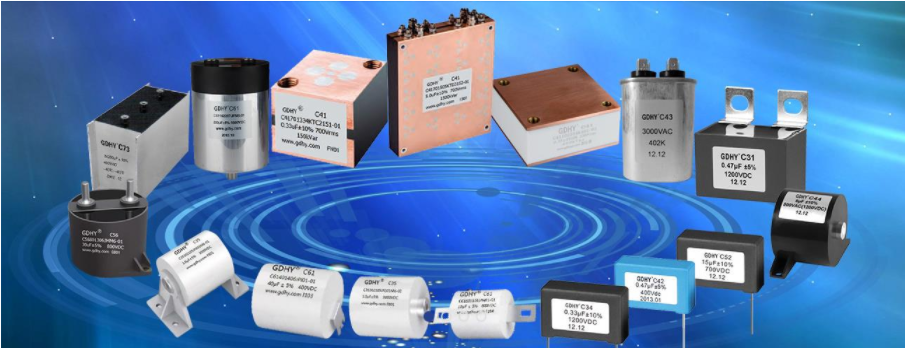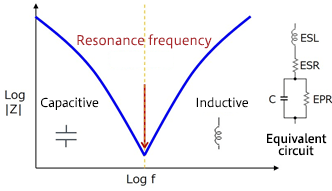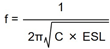The frequency characteristics of electronic film capacitors, relative to ESR and ESL
2022-12-20

Measures to address noise using capacitors and inductors, explaining what might be called the fundamentals of noise countermeasures. Here we use a simple four-element model. In order to represent resonances at still higher frequencies, models based on still more elements may be used.Understanding the frequency characteristics of capacitors

When using capacitors to handle noise problems, a good understanding of the capacitor characteristics is essential. This diagram shows the relationship between capacitor impedance and frequency, and is a characteristic that is basic to any capacitor.In additional to the electrostatic capacitance C of the capacitor, there are also the resistive component ESR (equivalent series inductance), and the EPR (equivalent parallel resistance), which exists in parallel with the electrostatic capacitance. The EPR means that there is an insulating resistance IR between electrodes,or that there is a leakage current between electrodes. It may be that the term IR is generally used.

C and ELS form a series resonance circuit, and the impedance of the capacitor has what is essentially a V-shape frequency characteristic, as shown in the diagram. Up until the resonance frequency, the capacitive characteristic is exhibited, and the impedance falls. The impedance at the resonance frequency depends on the ESR. When the resonance frequency is exceeded, the impedance characteristic changes to inductive, and as the frequency rises, the impedance increases. The inductive impedance characteristic depends on the ESL.

The resonance frequency can be calculated using this equation:This equation indicates that the smaller the electrostatic capacitance and the smaller the ESL of a capacitor, the higher is the resonance frequency. When applying this to the elimination of noise, a capacitor with a smaller capacitance and smaller ESL has a lower impedance at a higher frequency, and so is better for removing high-frequency noise.

The order of the explanation here is reversed, but noise countermeasures that employ capacitors make use of the basic capacitor characteristic of "passing AC currents, and passing them more easily at higher frequencies". Capacitors are thus used to shunt unwanted noise (AC components) away from signals or power supply lines to GND, for example.

The following graph shows the frequency characteristics of the impedance of capacitors with different electrostatic capacitances. In the capacitive characteristic region, the larger the capacitance, the lower is the impedance. Moreover, the smaller the capacitance, the higher is the resonance frequency, and the lower is the impedance in the inductive characteristic region.